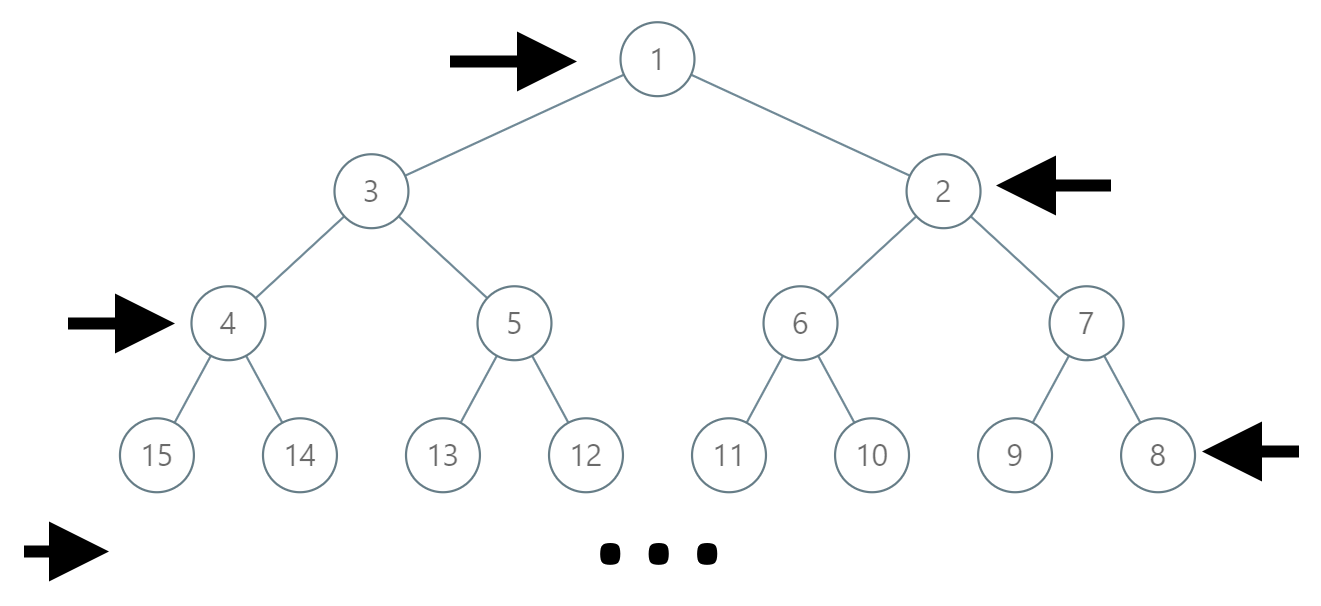Formatted question description: https://leetcode.ca/all/1104.html

# 1104. Path In Zigzag Labelled Binary Tree (Medium)

In an infinite binary tree where every node has two children, the nodes are labelled in row order.

In the odd numbered rows (ie., the first, third, fifth,...), the labelling is left to right, while in the even numbered rows (second, fourth, sixth,...), the labelling is right to left.Given the label of a node in this tree, return the labels in the path from the root of the tree to the node with that label.

Example 1:

Input: label = 14
Output: [1,3,4,14]


Example 2:

Input: label = 26
Output: [1,2,6,10,26]


Constraints:

• 1 <= label <= 10^6

Related Topics:
Math, Tree

## Solution 1.

// OJ: https://leetcode.com/problems/path-in-zigzag-labelled-binary-tree/

// Time: O(logN)
// Space: O(1)
class Solution {
inline int r(int label, int row) {
return 3 * (1 << row) - label - 1;
}
public:
vector<int> pathInZigZagTree(int label) {
vector<int> ans(1, label);
while (label != 1) {
int row = log2(label);
if (row % 2) label = r(label, row) / 2;
else label = r(label / 2, row - 1);
ans.push_back(label);
--row;
}
reverse(ans.begin(), ans.end());
return ans;
}
};


Java

class Solution {
public List<Integer> pathInZigZagTree(int label) {
Stack<Integer> stack = new Stack<Integer>();
while (label >= 1) {
stack.push(label);
int log = (int) (Math.log(label) / Math.log(2));
int minValue = (int) Math.pow(2, log);
label = minValue * 3 - 1 - label;
label /= 2;
}
List<Integer> path = new ArrayList<Integer>();
while (!stack.isEmpty())